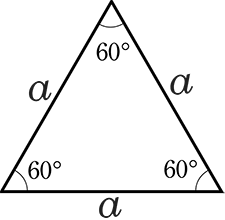Fill in here cm

### Remember !

Properties of the equilateral triangle.

The equilateral triangle has equal sides and angles and the angle is 60°

The perimeter of an equilateral triangle is equal to the sum of the sides.

The equilateral triangle has all the congruent midlines.

Abbreviations: A - equilateral triangle area and h - equilateral triangle height.

A =
a23/4

h =
a√3/2Site-ul nostru poate rămâne gratuit numai prin afișarea de reclame.

Vă rugăm să ne susțineți prin dezactivarea blocării de anunțuri.